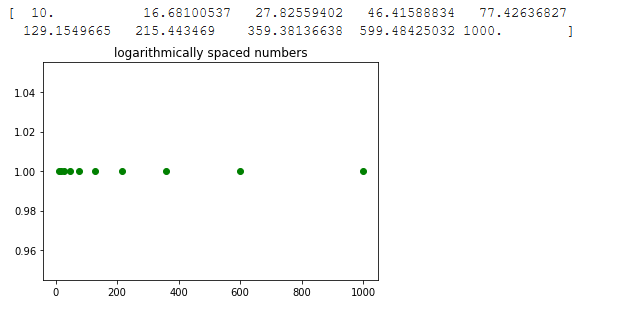Open in App
Not now

# How to create a list of uniformly spaced numbers using a logarithmic scale with Python?

• Last Updated : 15 Mar, 2021

In this article, we will create a list of uniformly spaced numbers using a logarithmic scale. It means on a log scale difference between two adjacent samples is the same. The goal can be achieved using two different functions from the Python Numpy library.

### Functions  Used:

• numpy.logspace: This function returns number scaled evenly on logarithmic scale.

Parameters:

• start:  Starting value of sequence is base**start
• stop: If endpoint is True then ending value of sequence is base**stop
• num (Optional): Specifies the number of samples to generate
• endpoint (Optional): It can either be true or false with default value true
• base (Optional): Specifies the base of log sequence. Default value is 10.
• dtype (Optional): Specifies the type of output array
• axis (Optional): The axis in the result to store the samples.

Return: It returns array of samples equally spaced on log scale.

• numpy.geomspace: This function is similar to logspace function only difference being end points are specified directly. In Output sample every output is obtained by multiplying previous output by same constant.

Parameters:

start:  It is the starting value of sequence

stop: If endpoint is True then it is the ending value of sequence

num (Optional): Specifies the number of samples to generate

endpoint (Optional): It can either be true or false with default value true

dtype (Optional): Specifies the type of output array

axis (Optional): The axis in the result to store the samples.

Return: It returns array of samples equally spaced on log scale.

Example 1: This example uses logspace function. In this example, start is passed as 1 and the stop is passed as 3 with the base being 10. So starting point of the sequence will be 10**1 = 10 and the ending point of the sequence will be 10**3 = 1000.

## Python3

 `# importing the library``import` `numpy as np``import` `matplotlib.pyplot as plt`` ` `# Initializing variable``y ``=` `np.ones(``10``)`` ` `# Calculating result``res ``=` `np.logspace(``1``, ``3``, ``10``, endpoint ``=` `True``)`` ` `# Printing the result``print``(res)`` ` `# Plotting the graph``plt.scatter(res, y, color ``=` `'green'``)``plt.title(``'logarithmically spaced numbers'``)``plt.show()`

Output:Example 2: This example generates the same list as the previous example using geomspace function. Here we directly passed 10 and 1000 as starting and ending points

## Python3

 `# importing the library``import` `numpy as np``import` `matplotlib.pyplot as plt`` ` `# Initializing variable``y ``=` `np.ones(``10``)`` ` `# Calculating result``res ``=` `np.geomspace(``10``, ``1000``, ``10``, endpoint ``=` `True``)`` ` `# Printing the result``print``(res)`` ` `# Plotting the graph``plt.scatter(res, y, color ``=` `'green'``)``plt.title(``'logarithmically spaced numbers'``)``plt.show()`

Output:Example 3: In this example, endpoint is set to false so it will generate n+1 sample and return only first n sample i.e. stop will not be included in the sequence.

## Python3

 `# importing the library``import` `numpy as np``import` `matplotlib.pyplot as plt`` ` `# Initializing variable``y ``=` `np.ones(``10``)`` ` `# Calculating result``res ``=` `np.logspace(``1``, ``3``, ``10``, endpoint ``=` `False``)`` ` `# Printing the result``print``(res)`

Output:

```[ 10.          15.84893192  25.11886432  39.81071706  63.09573445
100.         158.48931925 251.18864315 398.10717055 630.95734448]```

My Personal Notes arrow_drop_up